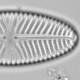## Doubly re-ordered data matrices

A useful method for exploring and visualising the main patterns in community data is a doubly reordered data matrix, with the species abundance indicated by either the size of a symbol or shading. In this example, I’ve used the dune species data that is built into the vegan library in R and ordered the sites (rows) and species (columns) by their axis 1 scores from a correspondence analysis. I could have re-ordered the sites by an environmental variable and the species by their weighted average optima on this gradient.```library(vegan)
data(dune)

mod<-cca(dune) # run a correspondence analysis with the data
dune<-dune[order(scores(mod,display="sites", choice=1)), order(scores(mod,display="species", choice=1))] # reorder the species data

par(mar=c(3,4,.5,.5), mgp=c(1.5,.5,0), tcl=-0.3)
plot(row(dune), col(dune), pch=20, cex=unlist(dune)/2,axes=FALSE, ann=FALSE)
axis(1, at=1:nrow(dune), labels=rownames(dune), las=2, cex.axis=.9)
axis(2, at=1:ncol(dune), labels=colnames(dune), las=2, cex.axis=.9)
```

The function row() takes a matrix or data.frame x and return a matrix the same dimensions as x with the first row all ones, the second all twos, and so on. The function col() does the equivalent for the columns.